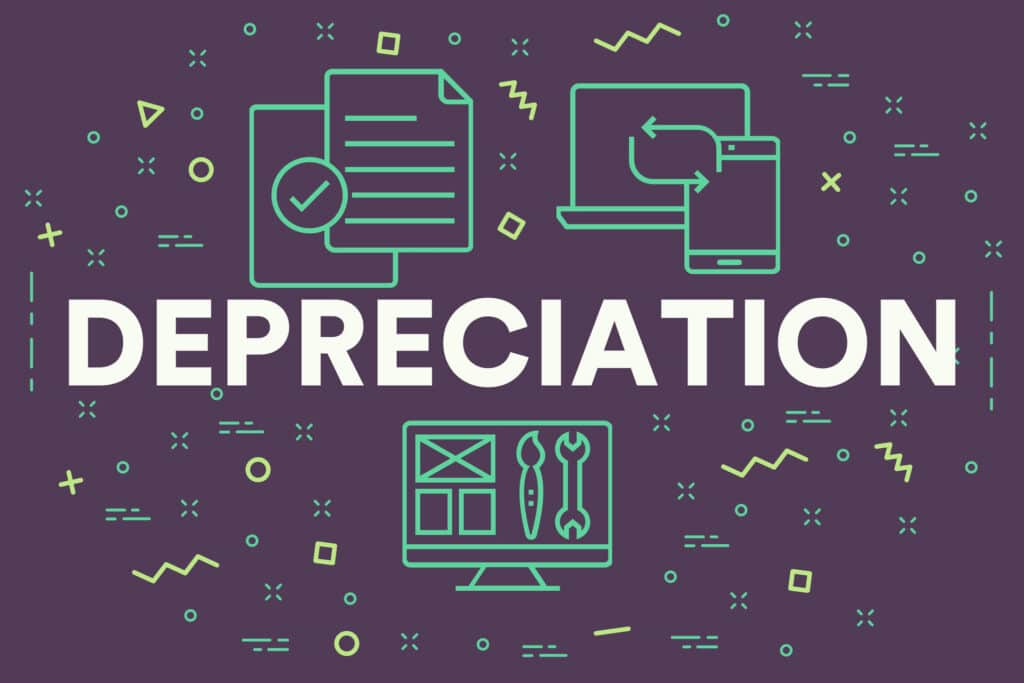# Depreciation in AccountingWritten by Team 365 financeDepreciation is a method in accountancy of sharing out the cost of an asset over its expected or useful life. Another way of understanding this is to think of it as showing how much of the value of an asset has already been used. It is important for a company to take depreciation into account because it can make an impact on profits.

Many of the assets bought and owned by a company are expensive. Using depreciation allows a company to spread the cost of the asset over time and generate revenue from it in the meantime. Depreciation takes account of the fact that the value of an asset will diminish over time.

Depreciation can be used by a company for tax purposes, too. Deductions for depreciation can be helpful to reduce the amount of income upon which tax is payable.

## How to Record Depreciation in Practice

When a company buys an asset, it will be recorded as an asset on an asset account and it will then be shown on the balance sheet. To balance the accounts, the purchase is shown as a credit on the cash or accounts payable side. The cost of the asset is moved to the income statement from the balance sheet by applying depreciation. The accountant will do this at the end of each accounting period for all assets which have not yet been subject to full depreciation. Each company will have its own threshold for when to begin depreciating assets.

To record the depreciation each accounting period, a debit is made to depreciation expenses and a credit allocated to accumulated depreciation, flowing through to the income statement and balance sheet respectively.

Accumulated depreciation on an asset is the total cumulative depreciation on that asset up to the point in question. The salvage value of an asset is the amount for which a company expects to be able to dispose of the asset when it reaches the end of its useful life.

## Examples of Depreciation

As an example of depreciation accounting, imagine that a company invests £50,000 in new machinery. This is a substantial sum to deal with within one year’s accounts. If the machinery is likely to have a useful life of 10 years, and can be sold for £10,000 at the end of the life span, it has a salvage value of £10,000.

The depreciation expense will be the difference between the initial cost, £50,000, and the salvage value, £10,000, divided by the useful life of 10 years. The calculation of depreciation expense is, therefore, £40,000 divided by 10, or £4,000 per year. This allows the company to spread the cost of the acquisition over the machinery’s useful life span, boosting net income in the meantime.

If an item of machinery used by a business initially cost £25,000 and has been depreciated by £10,000, its carrying value for the balance sheet is the historical cost of £25,000, less the depreciation of £10,000, therefore £15,000. Once all the available depreciation has been used up, the carrying value becomes known as the salvage value of the asset.

The total amount which is depreciated each year is called the depreciation rate. This is recorded as a percentage. As an example, if a company had £10,000 in depreciation over an asset’s expected life span and an annual depreciation of £1500, the depreciation rate would be 15% per annum.

## Types of Depreciation

There are different types of depreciation, used for different purposes in depreciation accounting.

### Accumulated depreciation

This the total of all depreciation recorded against an asset up to a specific date.

### Declining balance

This is a method of accelerated depreciation. It depreciates the asset at a straight-line depreciation percentage multiplied by its remaining depreciable amount. This causes a bigger depreciation amount in earlier years which then declines year on year.

### Straight line

This method allocates an equal sum of depreciation each year through the whole life span of the asset until the salvage value has been reached.

### Units of Production

The units of production depreciation calculation is based upon estimating the total units an asset will produce over its lifespan and then calculating each year’s depreciation based on the number of units produced in that accounting period.

As with many other accounting methods, there’s no “best” method of calculating depreciation for every business. In some cases, simple methods such as straight line depreciation may offer the best results for your business; in others, a more complicated method might be required.

## Summary

Like many other accounting concepts, depreciation can be difficult to wrap your head around at first. However, as one of the most important parts of maintaining reliable accounting records, it’s something that every business owner or investor should take time to learn.

By using depreciation, you’ll find it easier to accurately record the cost of purchasing and using company assets, helping you stay more aware of your business’s overall financial condition.

If your business requires funding, visit our How it Works page to see how our fast, affordable finance has helped thousands of SMEs in the UK.